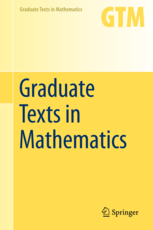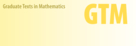## Series Monográficas: Graduate Texts in MathematicsVol. 30 Jacobson, Nathan
Lectures in abstract algebra: basic concepts
QA266 J3 1951

Vol. 15 Berberian, Sterling K.
Lectures in functional analysis and operator theory
QA322 B46

Commutative algebra II
QA251.3 Z37 1975

Vol. 14 Golubitsky, Martin
Stable mappings and their singularities
QA613 G64

Vol. 28 Zariski, Oscar; Samuel, Pierre
Commutative algebra I
QA251.3 Z37

Rings and categories of modules
QA247 A52

Vol. 27 Kelley, John L.
General topology
QA611 K42

Vol. 12 Beals, Richard
Advanced mathematical analysis : periodic functions and distributions complex analysis, laplace transform and applications
QA300 B42

Vol. 26
Algebraic theories
QA251 M324

Vol. 11 Conway, John B.
Functions of one complex variable 2nd ed.
QA331 C64 1978

Vol. 25 Hewitt, Edwin
Real and abstract analysis : A modern treatment of the theory of functions of a real variable
QA331.5 H4

Vol. 10 Cohen, M. M.
A course in simple homotopy theory
QA611 C622

Vol. 24 Holmes, Richard B.
Geometric functional analysis and its applications
QA320 H65

Vol. 9 Humphreys, James E.
Introduction to lie algebras and representation theory
QA251 H84

Vol. 23 Greub, Werner Hildbert
Linear algebra. 4th ed.
QA251 G73 1981

Vol. 8 Takeuti, Gaisi
Axiomatic set theory
QA248 T33

Vol. 22 Barnes, Donald W.; Mack, John M.
An algebraic introduction to mathematical logic
QA9 B36

Vol. 7  Serre, Jean Pierre
A course in arithmetic
QA243 S47

Vol. 21 Humphreys, James E.
Linear algebraic groups
QA171 H84

Projective planes
QA471 H84

Vol. 20 Husemöller, Dale
Fibre bundles 3rd ed.
QA612.6 H87 1994

Vol. 5 Mac Lane, Saunders
Categories for the working mathematician
QA169 M32

Vol. 19 Halmos, Paul R.
A Hilbert space problem book 2nd rev. and enl. ed.
QA322 H33 1982

Vol. 4 Hilton, Peter John; Stammbach, Urs
A course in homological algebra
QA169 H55

Vol. 18 Halmos, Paul R.
Measure theory
QA312 H35

Vol. 3 Schaefer, H. H.
Topological vector spaces. 2nd ed.
QA322 S23 1999

Vol. 17 Rosenblatt, Murray
Random processes 2nd ed.
QA274 R64 1974

Vol. 16  Winter, David J .
The structure of fields
QA247 W54

Vol. 1 Takeuti, Gaisi
Introduction to axiomatic set theory
QA248 T335

### ¿Quién está en línea?

Hay 121 invitados y ningún miembro en línea

Go to top